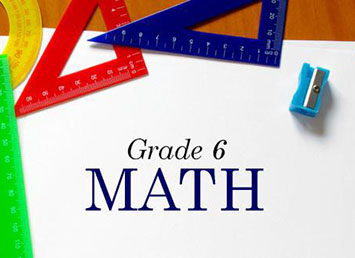FIND OUT MORE

SPOTLIGHT
FIND A COURSE Search Courses

Browse course catalogCOURSE DESCRIPTION6th grade math as an introductory middle level math course. Students will learn about statistical thinking and organizing data. Students also will learn about problem solving, exponents, and order of operations. Students will also learn basic Algebra and Geometry. By the end of this course students should also be able to perform operations using numbers other than whole numbers. This includes working with decimals, fractions, and mixed numbers. Students will learn to convert fractions to decimals and decimals to fractions. They should also understand how to multiply, divide, add, and subtract decimals, fractions, and mixed numbers.
CHAPTERS
 Ch.1 – Geometry Identify the Parts of a Solid Areas of Quadrilaterals & Triangles Parts of Geometric Figures Constructing Geometric Figures Areas of Polygons Circumference and Area of a Circle Similar Triangles Radius and Diameter Circumference and Area Identifying Parts of a Circle Ordered Pairs Areas on the Coordinate Plane Areas of Irregular Polygons Volume of a Rectangular Prism Supplementary, Complementary and Vertical Angles Geometry Test Ch.2 – Number System Operations on Mixed Numbers Using Positive and Negative Numbers to Describe Quantities Division of Fractions Using Positive and Negative Numbers to Describe Quantities Graphing Points Exponents: Prime Factorization Multiples and Least Common Multiples Factors and Greatest Common Factor Add, Subtract, Multiply and Divide Decimals Multi-Digit Division Identifying Factors Comparing and Ordering Numbers in Different Forms Integers: Addition and Subtraction Absolute Value Comparing and Ordering Numbers Estimating Percents Identifying Percents Reciprocals Operations on Mixed Numbers Rational Numbers on a Number Line Radicals: Inverse Relationships Exponential Form Coordinate Geometry Multiply Multi-Digit Decimals Fractions, Decimals and Percents Multiply and Divide Fractions Multiplying Decimals Dividing with Decimals Ch.3 – Expressions amd Equations Describing Relationships Using Independent and Dependent Variables Equivalent Expressions Proving Expressions are Equivalent Expressions Involving Exponents Describing Relationships Using Independent and Dependent Variables Proving Expressions are Equivalent Equivalent Expressions Expressions Involving Exponents Expressions with Exponents Translating Verbal Expressions into Inequalities Solving Two-Step Equations Writing Algebraic Expressions Solving One-Step Equations Translating Verbal Expressions Evaluate Expressions with Rational Numbers Order of Operations Translating Mathematical Sentences Expressions amd Equations Test Ch.4 – Statistics and Probability Statistical vs. Non-Statistical Questions Summary Statistics Median, Mode and Range Predicting Experiment Results Compare Summary Statistics Dependent Events Frequency Tables Counting Principle Interpreting Graphs Venn Diagrams Making Predictions Using Data Statistics and Probability Test